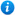# The Four Operations Challenge Cards - Year 6

The Four Operations Challenge Cards - Year 6

Year 6 maths programme of study - Number (addition, subtraction, multiplication and division):

• multiply multi-digit numbers up to 4 digits by a two-digit whole number using the formal written method of long multiplication
• divide numbers up to 4 digits by a two-digit number using the formal written method of short division where appropriate, interpreting remainders according to the context
• solve addition and subtraction multi-step problems in contexts, deciding which operations and methods to use and why
• use their knowledge of the order of operations to carry out calculations involving the four operations
• solve problems involving addition, subtraction, multiplication and division
These Year 6 maths challenge cards have been designed to consolidate pupils understanding of the curriculum objectives of the Year 6 maths programme of study listed above and compliment the Year 6 teaching resources listed below.
Related resources:

Click on the images from the PowerPoint presentation to view the resource in more detail.

Our Price : £1.00 / 1 Credits